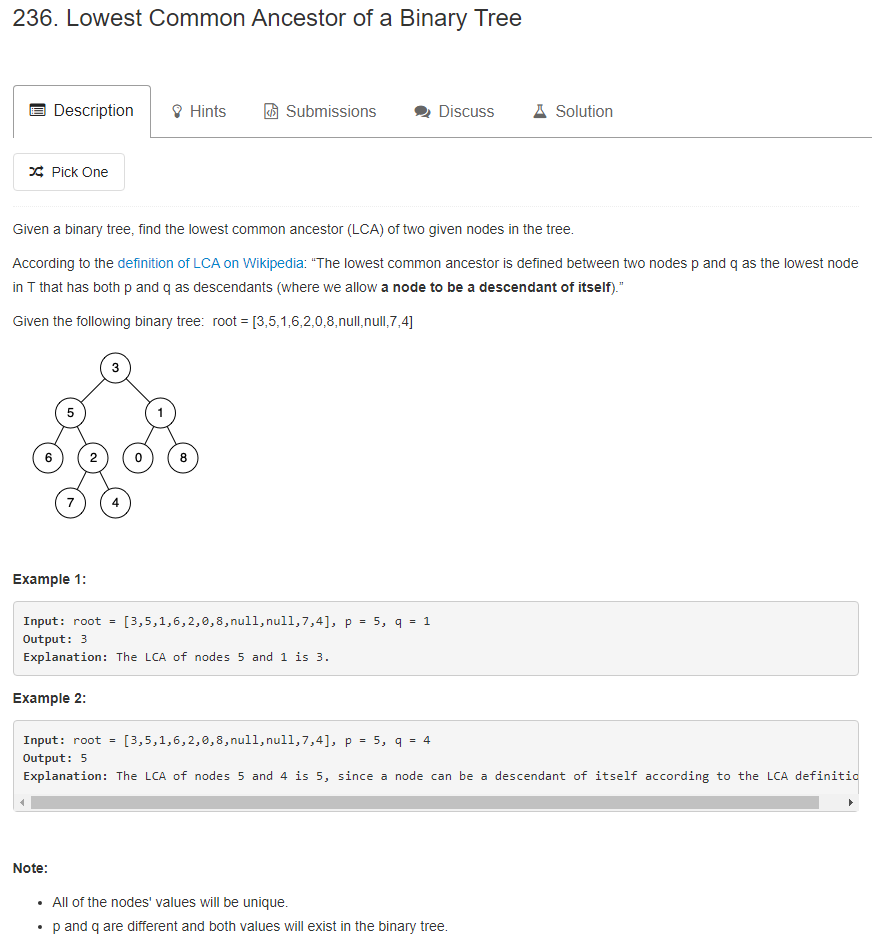# 题目描述（中等难度）# 解法一

• 如果给定的两个节点的值都小于根节点的值，那么最近的共同祖先一定在左子树
• 如果给定的两个节点的值都大于根节点的值，那么最近的共同祖先一定在右子树
• 如果一个大于等于、一个小于等于根节点的值，那么当前根节点就是最近的共同祖先了

public TreeNode lowestCommonAncestor(TreeNode root, TreeNode p, TreeNode q) {
if (root == p || root == q) {
return root;
}
Stack<TreeNode> stack = new Stack<>();
//中序遍历判断两个节点是否在左子树
TreeNode cur = root.left;
boolean pLeft = false;
boolean qLeft = false;
while (cur != null || !stack.isEmpty()) {
// 节点不为空一直压栈
while (cur != null) {
stack.push(cur);
cur = cur.left; // 考虑左子树
}
// 节点为空，就出栈
cur = stack.pop();
// 判断是否等于 p 节点
if (cur == p) {
pLeft = true;
}
// 判断是否等于 q 节点
if (cur == q) {
qLeft = true;
}

if(pLeft && qLeft){
break;
}
// 考虑右子树
cur = cur.right;
}

//两个节点都在左子树
if (pLeft && qLeft) {
return lowestCommonAncestor(root.left, p, q);
//两个节点都在右子树
} else if (!pLeft && !qLeft) {
return lowestCommonAncestor(root.right, p, q);
}
//一左一右
return root;
}


# 解法二

public TreeNode lowestCommonAncestor(TreeNode root, TreeNode p, TreeNode q) {
if (root == null || root == p || root == q ) {
return root;
}
TreeNode leftCommonAncestor =  lowestCommonAncestor(root.left, p, q);
TreeNode rightCommonAncestor =  lowestCommonAncestor(root.right, p, q);
//在左子树中没有找到，那一定在右子树中
if(leftCommonAncestor == null){
return rightCommonAncestor;
}
//在右子树中没有找到，那一定在左子树中
if(rightCommonAncestor == null){
return leftCommonAncestor;
}
//不在左子树，也不在右子树，那说明是根节点
return root;
}


# 解法三

root 节点一定是 p 节点和 q 节点的共同祖先，只不过这道题要找的是最近的共同祖先。

root 节点出发有一条唯一的路径到达 p

root 节点出发也有一条唯一的路径到达 q

       root
|
|
|
r
/ \
/   \
/    /
\    \
p    \
q


root -> * -> * -> r -> x -> x -> p

root -> * -> * -> r -> y -> y -> y -> y -> q

public TreeNode lowestCommonAncestor(TreeNode root, TreeNode p, TreeNode q) {
Stack<TreeNode> stack = new Stack<>();
HashMap<TreeNode, TreeNode> parent = new HashMap<>();
stack.push(root);
parent.put(root, null);
//将遍历过程中每个节点的父节点保存起来
while (!parent.containsKey(p) || !parent.containsKey(q)) {
TreeNode cur = stack.pop();
if (cur.left != null) {
stack.push(cur.left);
parent.put(cur.left, cur);
}
if (cur.right != null) {
stack.push(cur.right);
parent.put(cur.right, cur);
}
}
HashSet<TreeNode> path = new HashSet<>();
// 倒着还原 p 的路径，并将每个节点加入到 set 中
while (p != null) {
p = parent.get(p);
}

// 倒着遍历 q 的路径，判断是否在 p 的路径中
while (q != null) {
if (path.contains(q)) {
break;
}
q = parent.get(q);
}
return q;
}